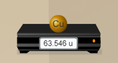# Moles

### DESCRIPTION

Understand the definition of a mole and determine the Avogadro constant by adding atoms or formula units to a balance until the mass in grams is equal to the atomic or formula mass. Manipulate a conceptual model to understand how the number of particles, the number of moles, and the mass are related. Then use dimensional analysis to convert between particles, moles, and mass.

Full Lesson Info